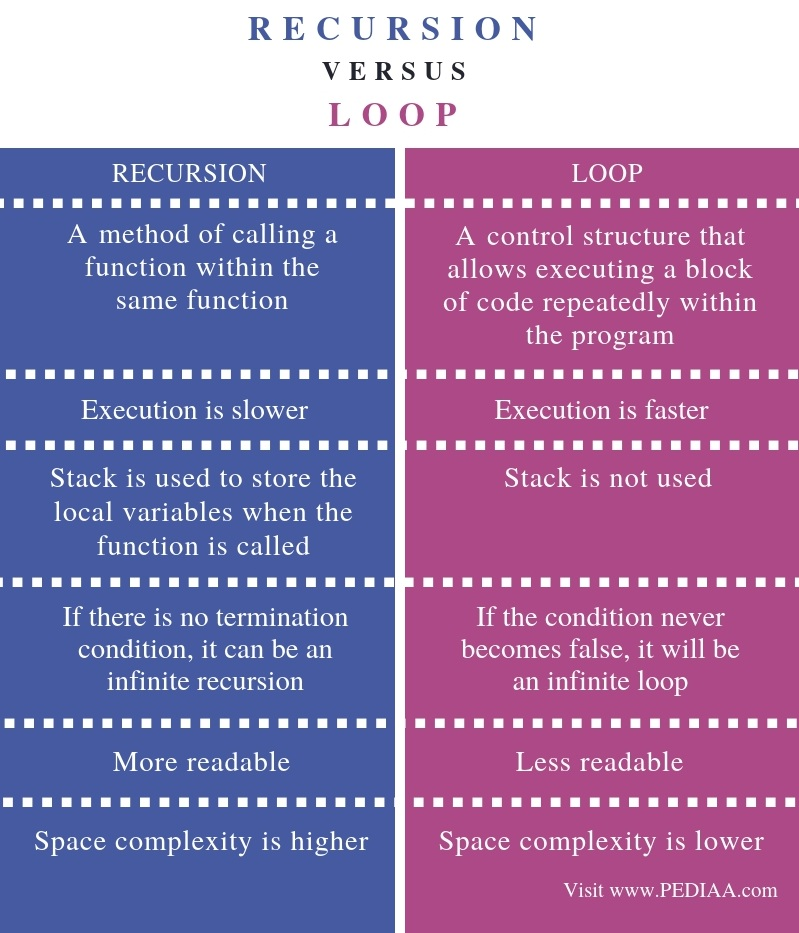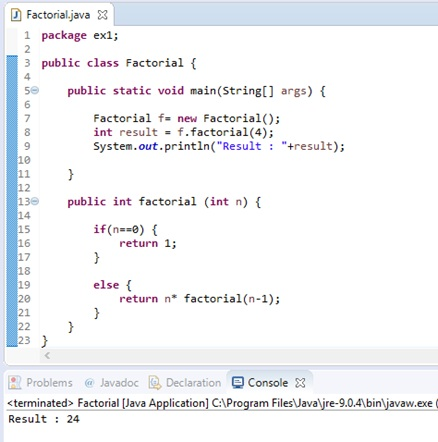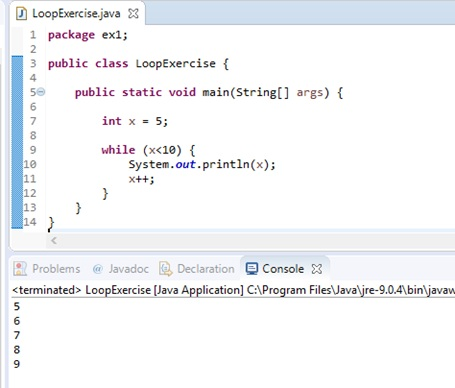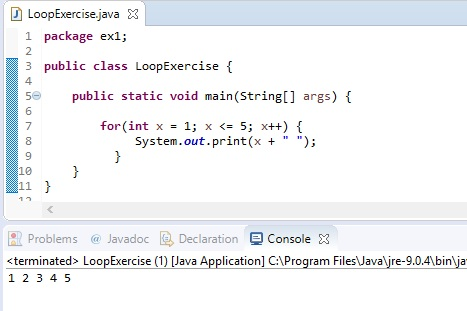# What is the Difference Between Recursion and Loop

The main difference between recursion and loop is that recursion is a mechanism to call a function within the same function while loop is a control structure that helps to execute a set of instructions again and again until the given condition is true.

Recursion and loop are two programming concepts. Both these techniques help to develop small to complex programs.

### Key Areas Covered

1. What is Recursion
– Definition, Functionality
2. What is Loop
– Definition, Functionality
3. What is the Difference Between Recursion and Loop
– Comparison of Key Differences

### Key Terms

Do While Loop, For Loop, Loop, Recursion, While Loop## What is Recursion

When a function calls itself within the function, it is called recursion. An example of a program with recursion is calculating factorial.

n! = n * (n-1)!, if n>0

n! =1, if n=0;Figure 1: Factorial Program in Java

According to the above program, it creates an object of Factorial. Then, using that object, it calls the method factorial. And, the method gets the value 4. Next, the else section executes. Following that it calls factorial (3). Then, the else section executes. It calls factorial (2). Next, the else section executes. It calls factorial (1), and the else section executes again. It calls factorial (0). Now n is 0. It returns 1. Finally, 1x2x3x4 =24 is returned, and that values are displayed on the screen. Likewise, a factorial function calls itself again and again.

## What is Loop

Sometimes it is necessary to execute a block of code repeatedly. Programming languages provide a control structure called loop to execute a set of instructions. Loop executes a statement inside the block one after the other. There are three types of loops as while loop, for loop and do while loop. Moreover, an iteration also refers to a loop.

### While Loop

A while loop contains a test expression. If that expression is true, the statements inside the while loop executes. At the end of the statements, it checks the test expression again. Then, this process repeats until the test expression becomes false. When the test expression is false, the while loop terminates. Then the control passes to the first statement after the while loop.Figure 2: Program with while loop

In the above program, x is 5. It is less than 10. Therefore, it will print. Then, the x value increments. Now, x is 6. It is also less than 10; hence, it will print. Then again the x value increments. Now x is 7. Thus, this process repeats. When x is 10, the condition is false, and the loop terminates.

### For Loop

For loop contains the initialization, test expression and update. The initialization expression executes once. Then, it evaluates the test expression. If it is true, the statements inside for loop executes. At the end of the loop, it evaluates the updated expression. Thus, this process repeats until the test expression is false. When it is false, for loop terminates. Then, the control passes to the next statement after for loop.Figure 3: Program with For Loop

In the above for loop, x value is 1. It is less than 5. So, the value will print. Then the x value increments by 1. Now the x value is 2. It is also less than 5. Therefore, it will print. Then again, the x value increments by 1. Now, x is 3. This process repeats. When x is 6, the test condition becomes false, and the loop terminates.

### Do While Loop

Do while loop is similar to while loop, but it checks the condition after executing the loop statements. Therefore, whether the condition is true or false, the loop executes at least once. Here, checking of the condition happens after the loop executes. If the condition is true, the loop statements will execute again. This process repeats until the condition becomes false.Figure 4: Program With Do While Loop

The x value is initially 5. The do while loop executes and prints the value 5. Then, x becomes 6. It is less than 10. So, 6 will print. Next, x becomes 7. It is also less than 10. And, this process repeats. When x is 9, the value prints. But, when x becomes 10, the condition becomes false. Therefore, the loop terminates.

For example, assume that x is initially 20. It will print 20. Then x will increment, and x becomes 21. The test condition is false. Therefore, it will terminate the loop. The value 20 is higher than 10, and the test condition is false. However, the loop executes once. Therefore, do while loop executes at least once.

## Difference Between Recursion and Loop

### Definition

Recursion is a method of calling a function within the same function. In contrast, loop is a control structure that allows executing a block of code repeatedly within the program. These definitions contain the fundamental difference between recursion and loop.

### Speed

Speed is a major difference between recursion and loop. Recursion execution is slower. However, loop executes faster than recursion.

### Stack

In recursion, the stack is used to store the local variables when the function is called. But, loop does not use stack.

### Condition

If there is no termination condition, it can be an infinite recursion. However, if the condition never becomes false, it will be an infinite loop. This is another difference between recursion and loop.

### Space Complexity

Moreover, the space complexity of the recursive program is higher than a loop.

Another difference between recursion and loop is that a program with recursion is more readable than a program with loops.

### Conclusion

The difference between recursion and loop is that recursion is a mechanism to call a function within the same function while loop is a control structure that allows executing a set of instructions again and again until the given condition is true.

##### Reference:

1. “Recursion (Computer Science).” Wikipedia, Wikimedia Foundation, 12 Sept. 2018, Available here.
2. “Java Loop Control.” Www.tutorialspoint.com, Available here.